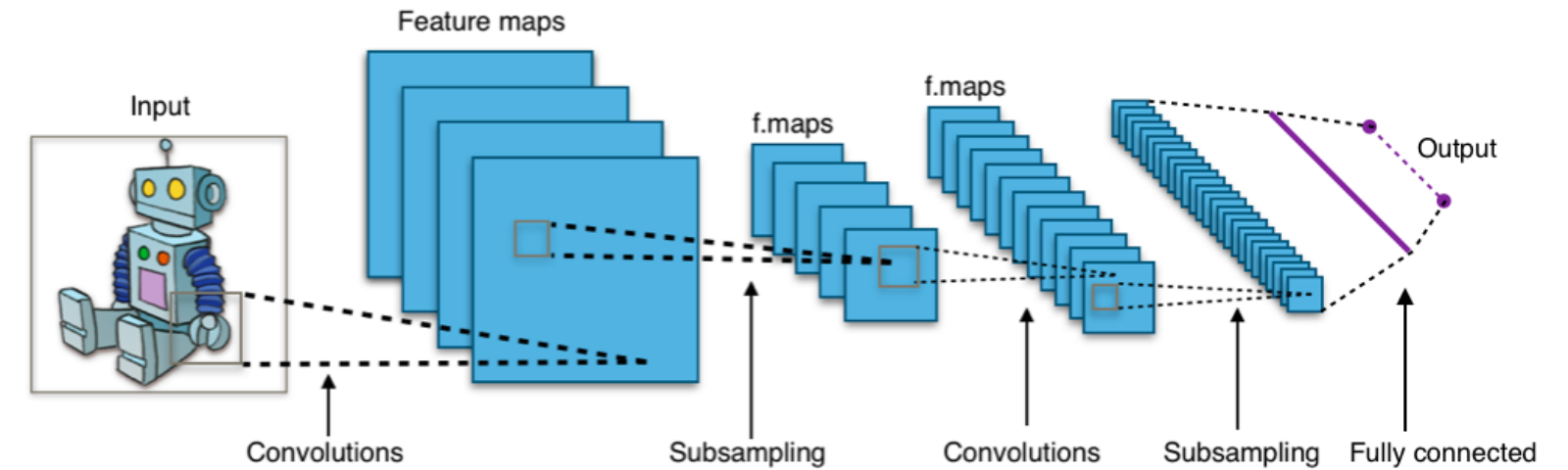# CNN (Convolutional Neural Network)

Original article was published by Anjani Suman on Deep Learning on Medium# CNN (Convolutional Neural Network)

Why CNN?

Convolution layers reduce the number of parameters and speed up the training of the model significantly.

CNN is basically a partially connected layer.

There are 3 types of layers in Convolutional Neural Network:

1. Convolutional Layer
2. Relu layer
3. Pooling Layer

Here I’m going to discuss all of 3 layers in detail:

1. Convolutional Layer:

The first layer on CNN is the convolutional layer. It applies a filter to input to create a feature map that summarizes the presence of detected features in the input.

In the convolutional layer, the image became the stack of the filtered images and the number of filtered images depends upon the number of filters.

2. Relu Layer:

In this layer, we remove all the negative values from the filtered images and replace them with zero’s.

3. Pooling layer:

The ultimate goal of the pooling layer is to reduce the size of the image.

Fully Connected Layer:

The fully connected layer, this is the layer where image classification actually happens.

Here we convert our filtered images into a 1-dimensional array.